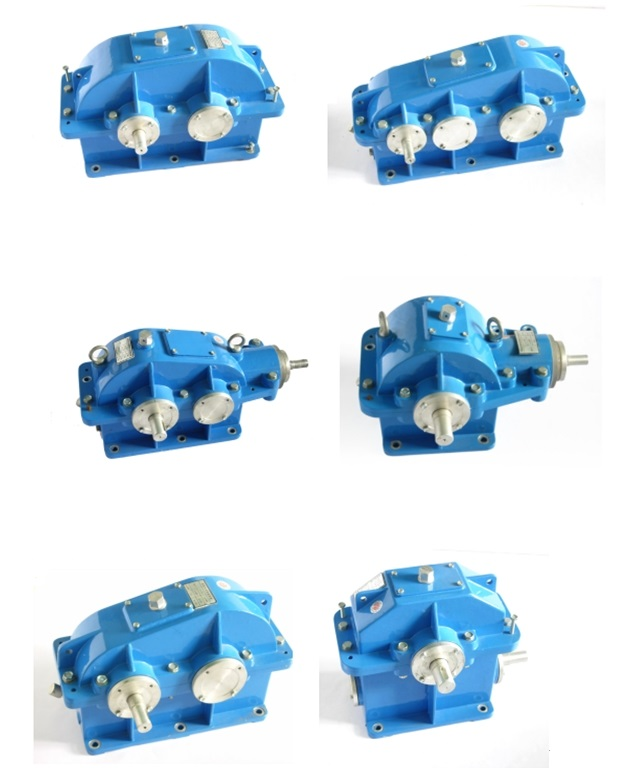15216837090当前位置：首页 > 产品中心 > > 机械教学模型 > 拆装用减速器实验室模型铝制|机械教学模型•# 拆装用减速器实验室模型铝制|机械教学模型

•更新时间：2023-05-17
•产品型号：
•厂商性质：生产厂家
•访  问  量：1184

Product Category

Related Articles

品牌 应用领域 育仰科教 文体,建材,汽车,综合

# 拆装用减速器实验室模型（铝制）名称 参数及尺寸 拆装用双级同轴式圆柱齿轮减速器 m=3Z1=22Z3=78Z2=26Z4=82ɑ=20°β=15°a=160.5i=11.1818h=159mm外形尺寸:560㎜×420㎜×350㎜重量:24.8KG（全部铝制） 拆装用双级展开式圆柱齿轮减速器 Z1=16Z2=47m=3Z3=27Z4=68а1=99ɑ=20°β15°а2=147i=7.398h=146mm外形尺寸:535㎜×330㎜×294㎜重量:21KG（全铝制） 拆装用双级圆锥圆柱齿轮减速器 m=3Z1=20Z2=50Z3=16Z4=68i=10.625h=141mma=130.5β=15°外形尺寸:550㎜×460㎜×360㎜重量:20KG（全部铝制） 拆装用单级圆锥齿轮减速器 m=3Z1=20Z2=60ɑ=20°β15°i=3h=129mm外形尺寸:500×350×315重量:15.5KG（全铝制） 拆装用单级圆柱齿轮减速器 m=3Z1=72Z2=29ɑ=20°β=15°a=158i=2.49h=151mm外形尺寸:450㎜×400㎜×340㎜重量:17.5KG（全部铝制） 拆装用蜗轮蜗杆减速器(下置式） z1=1q=12z2=45m=4㎜i=45H=210外形尺寸：420㎜×320㎜×400㎜重量15.5KG（全部铝制） 拆装用新型结构单级圆柱齿轮减速器 m=2Z1=25Z2=75i=3h=120外形尺寸：290㎜×260㎜×220㎜（全铝制） 拆装用双级分流式圆柱齿轮减速器 m=3Z1=16Z2=47Z3=27Z4=68i=7.398а1=99ɑ=20°h=146mmа2=147β15°外形尺寸:540㎜×360㎜×320㎜重量:24.5KG（全部铝制）

### 留言框

• #### 验证码：

请输入计算结果（填写阿拉伯数字），如：三加四=7地址：上海市松江区洞泾镇洞厍路601号邮箱：742805758@qq.com传真：021-60766769• 微信扫一扫